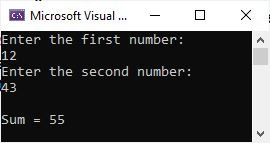# C# program to add two numbers

This post is published to add two numbers in C#. First, I will approach a program that does not receive any input from the user. Then I will create another program that will allow the user to input two numbers, which will be added, and the addition result will be printed back on the output console.

## Simple C# code to add two numbers

First, let me create a simple C# program that will add two numbers.

```int a = 10;
int b = 20;
int sum = a + b;

Console.WriteLine("Sum = " + sum);```

The output should exactly be:

`Sum = 30`

The same program can also be created in this way.

```int a = 10, b = 20;

Console.WriteLine("Sum = {0}", a + b);```

Caution: If you use "Sum = " + a + b,"  then both a and b will be treated as strings.

## C# code to add two numbers entered by user

Now let me create another C# program that will add and print the addition result of two numbers entered by the user at run-time of the program.

```Console.WriteLine("Enter the first number: ");
Console.WriteLine("Enter the second number: ");

Console.WriteLine("\nSum = {0}", a + b);```

The snapshot given below shows the sample run of this C# example after providing the user input of 12 and 43 as two numbers.I already defined how receiving inputs from a user occurs in C# on the main page of the C# tutorial, which you can easily find.

C# Online Test

« CodesCracker.com Next Program »